# 欢迎关注WX公众号：【程序员管小亮】

## 专栏C++学习笔记

《C++ Primer》学习笔记/习题答案 总目录

——————————————————————————————————————————————————————

# 第9章 - 顺序容器

## 练习9.1

• (a) 读取固定数量的单词，将它们按字典序插入到容器中。我们将在下一章中看到，关联容器更适合这个问题。
• (b) 读取未知数量的单词，总是将单词插入到末尾。删除操作在头部进行。
• (c) 从一个文件读取未知数量的整数。将这些数排序，然后将它们打印到标准输出。

（a）“按字典序插入到容器中” 意味着进行插入排序操作，从而需要在容器内部频繁进行插入操作，vector 在尾部之外的位置插入和删除元素很慢，deque 在头尾之外的位置插入和删除元素很慢，而 list 在任何位置插入、删除速度都很快。因此，这个任务选择 list 更为适合。当然，如果不是必须边读取单词边插入到容器中，可以使用 vector，将读入的单词依次追加到尾部，读取完毕后，调用标准库到排序算法将单词重排为字典序。

（b）由于需要在头、尾分别进行插入、删除操作，因此将 vector 排除在外，dequelist 都可以达到很好的性能。如果还需要频繁进行随机访问，则 deque 更好。

（c）由于整数占用空间很小，且快速的排序算法需频繁随机访问元素，将 list 排除在外。由于无须在头部进行插入、删除操作，因此使用 vector 即可，无须使用 deque

## 练习9.2

list<deque<int>> a;


## 练习9.3

• 两个迭代器 beginend 必须指向同一个容器中的元素，或者是容器最后一个元素之后的位置；
• 而且，对 begin 反复进行递增操作，可保证到达 end，即 end 不在 begin 之前。

## 练习9.4

#include<iostream>
#include<vector>
using namespace std;
bool search_vec(vector<int>::iterator beg,
vector<int>::iterator end, int val){
for (; beg != end; beg++){
if (*beg == val)
return true;
}
return false;
}
int main(){
vector<int> ilist = { 1, 2, 3, 4, 5, 6, 7 };
cout << search_vec(ilist.begin(), ilist.end(), 3) << endl;
cout << search_vec(ilist.begin(), ilist.end(), 8) << endl;
system("pause");
return 0;
}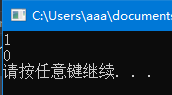## 练习9.5

#include<iostream>
#include<vector>
using namespace std;
vector<int>::iterator search_vec(vector<int>::iterator beg,
vector<int>::iterator end, int val){
for (; beg != end; beg++){
if (*beg == val)
return beg;
}
return end;
}
int main(){
vector<int> ilist = { 1, 2, 3, 4, 5, 6, 7 };
cout << search_vec(ilist.begin(), ilist.end(), 3) - ilist.begin() << endl;
cout << search_vec(ilist.begin(), ilist.end(), 8) - ilist.begin() << endl;
system("pause");
return 0;
}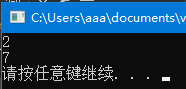## 练习9.6

list<int> lst1;
list<int>::iterator iter1 = lst1.begin(),
iter2 = lst1.end();
while (iter1 < iter2) /* ... */


vectordeque 不同，list 的迭代器不支持 < 运算，只支持递增、递减、= 以及 != 运算。

## 练习9.9

begincbegin 两个函数有什么不同？

cbegin 是C++新标准引入的，用来与 auto 结合使用。它返回指向容器第一个元素的 const 迭代器，可以用来只读地访问容器元素，但不能对容器元素进行修改。因此，当不需要写访问时，应该使用 cbegin

begin 则是被重载过的，有两个版本：其中一个是 const 成员函数，也返回 const 迭代器；另一个则返回普通迭代器，可以对容器元素进行修改。

## 练习9.10

vector<int> v1;
const vector<int> v2;
auto it1 = v1.begin(), it2 = v2.begin();
auto it3 = v1.cbegin(), it4 = v2.cbegin();


v1intvector 类型，我们可以修改 v1 的内容，包括添加、删除元素及修改元素值等操作。

v2int 的常量 vector 类型，其内容不能修改，添加、删除元素及修改元素值等均不允许。

beginauto 结合使用时，会根据调用对象的类型来决定迭代器的类型，因此 it1 是普通迭代器，可对容器元素进行读写访问，而 it2const 迭代器，不能对容器元素进行写访问。

cbegin 则不管调用对象是什么类型，始终返回 const 迭代器，因此 it3it4 都是 const 迭代器。

## 练习9.11

vector<int> vec;    // 0
vector<int> vec(10);    // 10个0
vector<int> vec(10, 1);  // 10个1
vector<int> vec{ 1, 2, 3, 4, 5 }; // 1, 2, 3, 4, 5
vector<int> vec(other_vec); // 拷贝 other_vec 的元素
vector<int> vec(other_vec.begin(), other_vec.end()); // 拷贝 other_vec 的元素


（1）vector<int>ilist1; //默认初始化，vector为空

size 返回0，表明容器中尚未有元素；capacity返回0，意味着尚未分配存储空间。这种初始化方式适合于元素个数和值未知，需要在程序运行中动态添加的情况。

（2）vector<int>ilist2(ilist); //ilist2初始化为ilist的拷贝

ilist 必须与 ilist2 类型相同，即也是 intvector 类型，ilist2 将具有与 ilist 相同的容量和元素。

vector<int>ilist21=ilist; //等价方式

（3）vector<int>ilist={1, 2, 3.0, 4, 5, 6, 7}; //ilist初始化为列表中元素的拷贝

vector<int>ilist_1{1, 2, 3.0, 4, 5, 6, 7}; //等价方式

（4）vector<int>ilist3(ilist.begin()+2, ilist.end()-1); //ilist3初始化为两个迭代器指定范围中的元素的拷贝

（5）vector<int>ilist4(7); //默认值初始化，ilist4中将包含7个元素

（6）vector<int>ilist5(7, 3); //指定值初始化，ilist5被初始化为包含7个值为3的int

## 练习9.13

#include<iostream>
#include<vector>
#include<list>
using namespace std;
int main()
{
list<int> ilist = { 1, 2, 3, 4, 5, 6, 7 };
vector<int> ivec = { 7, 6, 5, 4, 3, 2, 1 };
// 容器类型不同，不能使用拷贝初始化
//vector<double> ivec(ilist);
// 元素类型相容，因此可采用范围初始化
vector<double> dvec(ilist.begin(), ilist.end());
// 容器类型不同，不能使用拷贝初始化
// vector<double> dvecl(ivec);
// 元素类型相容，因此可采用范围初始化
vector<double> dvecl(ivec.begin(), ivec.end());

cout << dvec.capacity() << " " << dvec.size() << " "
<< dvec << " " << dvec[dvec.size() - 1] << endl;
cout << dvecl.capacity() << " " << dvecl.size() << " "
<< dvecl << " " << dvecl[dvecl.size() - 1] << endl;

system("pause");
return 0;
}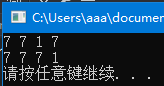## 练习9.14

#include<iostream>
#include<string>
#include<vector>
#include<list>
using namespace std;
int main()
{
list<char*> slist = { "hello", "world", "!" };
vector<string> svec;

// 容器类型不同，不能直接赋值
//	svec = slist;
// 元素类型相容，可采用范围初始化
svec.assign(slist.begin(), slist.end());

cout << svec.capacity() << " " << svec.size() << " " <<
svec << " " << svec[svec.size() - 1] << endl;

system("pause");
return 0;
}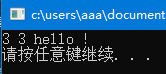## 练习9.15

#include<iostream>
#include<vector>
#include<list>
using namespace std;
int main()
{
vector<int> ivec = { 1, 2, 3, 4, 5, 6, 7 };
vector<int> ivec1 = { 1, 2, 3, 4, 5, 6, 7 };
vector<int> ivec2 = { 1, 2, 3, 4, 5 };
vector<int> ivec3 = { 1, 2, 3, 4, 5, 6, 8 };
vector<int> ivec4 = { 1, 2, 3, 4, 5, 7, 6 };

cout << (ivec == ivec1) << endl;
cout << (ivec == ivec2) << endl;
cout << (ivec == ivec3) << endl;
cout << (ivec == ivec4) << endl;

ivec.push_back(8);
ivec1.pop_back();
cout << ivec.capacity() << " " << ivec.size() << endl;
cout << (ivec == ivec1) << endl;

system("pause");
return 0;
}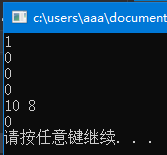## 练习9.16

    std::list<int>      li{ 1, 2, 3, 4, 5 };
std::vector<int>    vec2{ 1, 2, 3, 4, 5 };
std::vector<int>    vec3{ 1, 2, 3, 4 };

std::cout << (std::vector<int>(li.begin(), li.end()) == vec2 ? "true" : "false") << std::endl;
std::cout << (std::vector<int>(li.begin(), li.end()) == vec3 ? "true" : "false") << std::endl;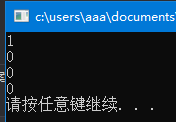## 练习9.17

	if (c1 < c2)


## 练习9.18

#include<iostream>
#include<deque>
#include<string>
using namespace std;
int main()
{
deque<string> sd;
string word;
while (cin >> word)
{
sd.push_back(word);
}
for (auto si = sd.cbegin(); si != sd.cend(); si++){
cout << *si << endl;
}

system("pause");
return 0;
}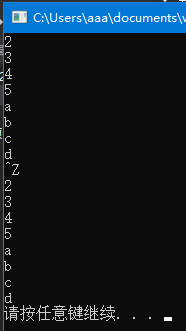## 练习9.19

list 来说，在任何位置添加新元素都有很好的性能，遍历操作也能高效完成，因此程序与上一题并无太大差异。

#include<iostream>
#include<list>
#include<string>
using namespace std;
int main()
{
list<string> sl;
string word;
while (cin >> word)
{
sl.push_back(word);
}
for (auto si = sl.cbegin(); si != sl.cend(); si++){
cout << *si << endl;
}

system("pause");
return 0;
}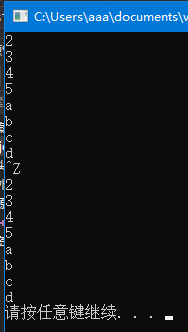## 练习9.20

#include<iostream>
#include<deque>
#include<list>
#include<string>
using namespace std;
int main()
{
list<int> ilist = { 1, 2, 3, 4, 5, 6, 7, 8 };
deque<int> odd_d, even_d;

for (auto iter = ilist.cbegin(); iter != ilist.cend();iter++){
if (*iter & 1){
odd_d.push_back(*iter);
}
else{
even_d.push_back(*iter);
}
}
for (auto iter = odd_d.cbegin(); iter != odd_d.cend(); iter++){
cout << *iter << " ";
}
cout << endl;
for (auto iter = even_d.cbegin(); iter != even_d.cend(); iter++){
cout << *iter << " ";
}
cout << endl;

system("pause");
return 0;
}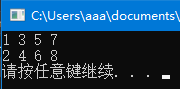## 练习9.21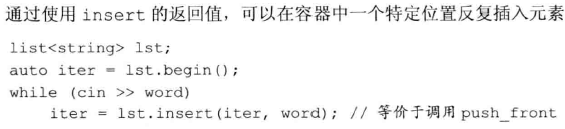#include<iostream>
#include<vector>
#include<string>
using namespace std;
int main()
{
vector<string> svec;
string word;
auto iter = svec.begin();
while (cin >> word)
{
svec.insert(iter, word);
}
for (auto iter = svec.cbegin(); iter != svec.cend(); iter++){
cout << *iter << endl;
}

system("pause");
return 0;
}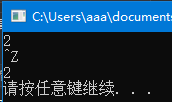## 练习9.22

vector<int>::iterator iter = iv.begin(),
mid = iv.begin() + iv.size() / 2;
while (iter != mid)
if (*iter == some_val)
iv.insert(iter, 2 * some_val);


#include<iostream>
#include<vector>
#include<string>
using namespace std;
int main()
{
vector<int> iv = { 1, 1, 2, 1 };
int some_val = 1;
vector<int>::iterator iter = iv.begin();
int org_size = iv.size(), new_ele = 0;
while (iter != (iv.begin() + org_size / 2 + new_ele))
{
if (*iter == some_val){
iter = iv.insert(iter, 2 * some_val);
new_ele++;
iter++; iter++;
}
else{
iter++;
}
}
for (iter = iv.begin(); iter != iv.end(); iter++){
cout << *iter << endl;
}

system("pause");
return 0;
}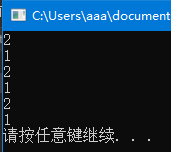## 练习9.23

4个变量的值会一样，都等于容器中唯一一个元素的值。

## 练习9.24

#include <iostream>
#include <vector>
using namespace std;
int main()
{
vector<int> iv;
cout << iv.at(0);
cout << iv;
cout << iv.front();
cout << *(iv.begin());

system("pause");
return 0;
}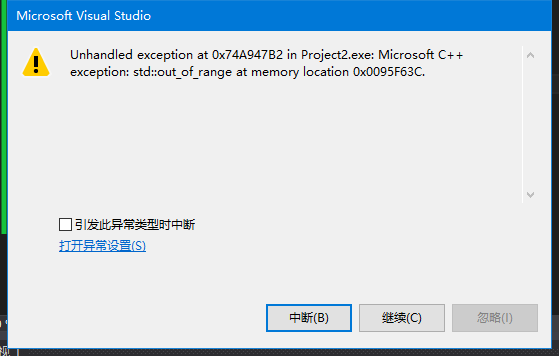## 练习9.26

int ia[] = { 0, 1, 1, 2, 3, 5, 8, 13, 21, 55, 89 };


#include<iostream>
#include<vector>
#include<list>
#include<string>
using namespace std;
int main()
{
int ia[] = { 0, 1, 1, 2, 3, 5, 8, 13, 21, 55, 89 };
vector<int> iv;
list<int> il;

iv.assign(ia, ia + 11);
il.assign(ia, ia + 11);
vector<int>::iterator iiv = iv.begin();
while (iiv != iv.end())
{
if (!(*iiv & 1)){
iiv = iv.erase(iiv);
}
else
iiv++;
}
list<int>::iterator iil = il.begin();
while (iil != il.end())
{
if (*iil & 1){
iil = il.erase(iil);
}
else
iil++;
}
for (iiv = iv.begin(); iiv != iv.end(); iiv++){
cout << *iiv << " ";
}
cout << endl;
for (iil = il.begin(); iil != il.end(); iil++){
cout << *iil << " ";
}
cout << endl;
system("pause");
return 0;
}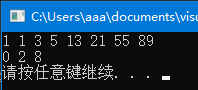## 练习9.27

#include <iostream>
#include <forward_list>
using namespace std;
int main()
{
forward_list<int> iflst = { 1, 2, 3, 4, 5, 6, 7, 8 };
auto prev = iflst.before_begin();
auto curr = iflst.begin();

while (curr != iflst.end())
{
if (*curr & 1){
curr = iflst.erase_after(prev);
}
else{
prev = curr;
++curr;
}
}
for (curr = iflst.begin(); curr != iflst.end(); curr++){
cout << *curr << " ";
}
cout << endl;
system("pause");
return 0;
}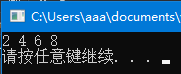## 练习9.28

#include <iostream>
#include <string>
#include <forward_list>
using namespace std;
void test_and_insert(forward_list<string> &sflst, const string &s1, const string &s2)
{
auto prev = sflst.before_begin();
auto curr = sflst.begin();
bool inserted = false;
while (curr != sflst.end())
{
if (*curr == s1)
{
curr = sflst.insert_after(curr, s2);
inserted = true;
}
prev = curr;
++curr;
}
if (!inserted){
sflst.insert_after(prev, s2);
}
}
int main()
{
forward_list<string> sflst = { "Hello", "!", "world", "!" };
test_and_insert(sflst, "Hello", "你好");
for (auto curr = sflst.begin(); curr != sflst.end(); curr++){
cout << *curr << " ";
}
cout << endl;
test_and_insert(sflst, "!", "?");
for (auto curr = sflst.begin(); curr != sflst.end(); curr++){
cout << *curr << " ";
}
cout << endl;
test_and_insert(sflst, "Bye", "再见");
for (auto curr = sflst.begin(); curr != sflst.end(); curr++){
cout << *curr << " ";
}
cout << endl;
system("pause");
return 0;
}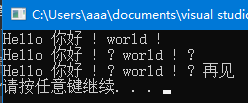## 练习9.31

listforward_list 与其他容器的一个不同是，迭代器不支持加减运算，究其原因，链表中元素并非在内存中连续存储，因此无法通过地址的加减在元素间远距离移动。因此，应多次调用++来实现与迭代器加法相同的效果。

#include <iostream>
#include <list>
using namespace std;
int main()
{
list<int> ilst = { 0, 1, 2, 3, 4, 5, 6, 7, 8, 9 };
auto curr = ilst.begin();

while (curr != ilst.end())
{
if (*curr & 1){
curr = ilst.insert(curr, *curr);
curr++; curr++;
}
else{
curr = ilst.erase(curr);
}
}
for (curr = ilst.begin(); curr != ilst.end(); curr++){
cout << *curr << " ";
}
cout << endl;
system("pause");
return 0;
}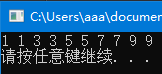#include <iostream>
#include <forward_list>
using namespace std;
int main()
{
forward_list<int> iflst = { 0, 1, 2, 3, 4, 5, 6, 7, 8, 9 };
auto prev = iflst.before_begin();
auto curr = iflst.begin();

while (curr != iflst.end())
{
if (*curr & 1){
curr = iflst.insert_after(curr, *curr);
prev = curr;
curr++;
}
else{
curr = iflst.erase_after(prev);
}
}
for (curr = iflst.begin(); curr != iflst.end(); curr++){
cout << *curr << " ";
}
cout << endl;
system("pause");
return 0;
}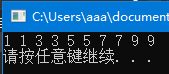## 练习9.32

iter = vi.insert(iter, *iter++);


## 练习9.33

vector 中插入新元素后，原有迭代器都会失效。因此，不将 insert() 返回的迭代器赋予 begin，会使 begin 失效。继续使用 begin 会导致程序崩溃。对此程序，保存尾后迭代器和不向 begin 赋值两个错误存在其一，程序都会崩溃。

## 练习9.34

iter = vi.begin();
while (iter != vi.end())
if (*iter % 2)
iter = vi.insert(iter, *iter);
++iter;


1. 若容器的第一个元素是偶数，布尔表达式为假，if 语句真分支不会被执行，iter 保持不变。循环继续执行，真分支仍然不会执行，iter 继续保持不变，如此陷入死循环。
2. 若容器的第一个元素是奇数，insert 语句被调用，将该值插入到首元素之前，并将返回的迭代器（指向新插入元素）赋予 iter，因此 iter 指向新首元素。继续执行循环，会继续将首元素复制到容器首位置，并令 iter 指向它，如此陷入死循环。

• 初始：{1，2，3，4，5，6，7，8，9}
• 第一步：{1，1，2，3，4，5，6，7，8，9}
• 第二步：{1，1，1，2，3，4，5，6，7，8，9}

## 练习9.35

capacity 返回已经为 vector 分配了多大内存空间（单位是元素大小），也就是在不分配新空间的情况下，容器可以保存多少个元素。

size 则返回容器当前已经保存了多少个元素。

## 练习9.37

list 是链表，当有新元素加入时，会从内存空间中分配一个新节点保存它；当从链表中删除元素时，该节点占用的内存空间会被立刻释放。因此，一个链表占用的内存空间总是与它当前保存的元素所需空间相等（换句话说，capacity 总是等于 size）。

array 是固定大小数组，内存一次性分配，大小不变，不会变化。

## 练习9.38

#include <iostream>
#include <string>
#include <vector>
using namespace std;
int main()
{
vector<int> vi;
int s, c;

for (s = vi.size(), c = vi.size(); s <= c; s++){
vi.push_back(1);
}
cout << "capacity: " << vi.capacity() << "  size: " << vi.size() << endl;
for (s = vi.size(), c = vi.size(); s <= c; s++){
vi.push_back(1);
}
cout << "capacity: " << vi.capacity() << "  size: " << vi.size() << endl;
for (s = vi.size(), c = vi.size(); s <= c; s++){
vi.push_back(1);
}
cout << "capacity: " << vi.capacity() << "  size: " << vi.size() << endl;
for (s = vi.size(), c = vi.size(); s <= c; s++){
vi.push_back(1);
}
cout << "capacity: " << vi.capacity() << "  size: " << vi.size() << endl;
for (s = vi.size(), c = vi.size(); s <= c; s++){
vi.push_back(1);
}
cout << "capacity: " << vi.capacity() << "  size: " << vi.size() << endl;
for (s = vi.size(), c = vi.size(); s <= c; s++){
vi.push_back(1);
}
cout << "capacity: " << vi.capacity() << "  size: " << vi.size() << endl;
for (s = vi.size(), c = vi.size(); s <= c; s++){
vi.push_back(1);
}
cout << "capacity: " << vi.capacity() << "  size: " << vi.size() << endl;
for (s = vi.size(), c = vi.size(); s <= c; s++){
vi.push_back(1);
}
cout << "capacity: " << vi.capacity() << "  size: " << vi.size() << endl;
for (s = vi.size(), c = vi.size(); s <= c; s++){
vi.push_back(1);
}
cout << "capacity: " << vi.capacity() << "  size: " << vi.size() << endl;
for (s = vi.size(), c = vi.size(); s <= c; s++){
vi.push_back(1);
}
cout << "capacity: " << vi.capacity() << "  size: " << vi.size() << endl;
system("pause");
return 0;
}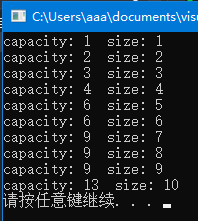## 练习9.39

vector<string> svec;
svec.reserve(1024);
string word;
while (cin >> word)
svec.push_back(word);
svec.resize(svec.size() + svec.size() / 2);


## 练习9.40

• 若读入了256个词，则 resize 之后容器的 capacity 将是384。
• 若读入了512个词，则 resize 之后容器的 capacity 将是768。
• 若读入了1000个词，则 resize 之后容器的 capacity 将是2048。
• 若读入了1048个词，则 resize 之后容器的 capacity 将是2048。

## 练习9.41

vector 提供了 data 成员函数，返回其内存空间的首地址。将此返回值作为 string 的构造函数的第一个参数，将 vectorsize 返回值作为第二个参数，即可获取 vector<char> 中的数据，将其看作一个字符数组来初始化 string

#include <iostream>
#include <string>
#include <vector>
using namespace std;
int main()
{
vector<char> vc = { 'H', 'E', 'L', 'L', 'O' };
string s(vc.data(), vc.size());
cout << s << endl;

system("pause");
return 0;
}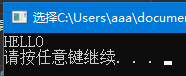## 练习9.42

#include <iostream>
#include <string>
#include <vector>
using namespace std;
void input_string(string &s){
s.reserve(100);
char c;
while (cin >> c)
{
s.push_back(c);
}
}
int main()
{
string s;
input_string(s);
cout << s << endl;

system("pause");
return 0;
}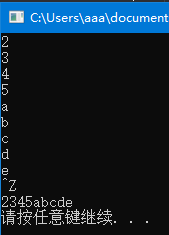## 练习9.43

1. 用迭代器 iter 遍历字符串 s。注意，对于 s 末尾少于 oldVal 长度的部分，己不可能与 oldVal 相等，因此无须检查。
2. 对每个位置，用一个循环检查 s 中字符是否与 oldVal 中的字符都相等。
3. 若循环是因为 iter2==oldVal.end 而退出，表明 siter 开始的子串与 oldVal 相等。则调用 erase 将此子串删除，接着用一个循环将 newVal 复制到当前位置。由于 insert 将新字符插入到当前位置之前，并返回指向新字符的迭代器，因此，逆序插入 newVal 字符即可。最后将 iter 移动到新插入内容之后，继续遍历 s
4. 否则，iter 开始的子串与 oldVal 不等，递增 iter，继续遍历 s
#include <iostream>
#include <string>
#include <vector>
using namespace std;
void replace_string(string &s, const string &oldVal, const string &newVal)
{
auto l = oldVal.size();
if (!l){
return;
}
auto iter = s.begin();
while (iter <= s.end() - 1)
{
auto iter1 = iter;
auto iter2 = oldVal.begin();
while (iter2 != oldVal.end() && *iter1 == *iter2)
{
iter1++;
iter2++;
}
if (iter2 == oldVal.end()){
iter = s.erase(iter, iter1);
if (newVal.size()){
iter2 = newVal.end();
do{
iter2--;
iter = s.insert(iter, *iter2);
} while (iter2 > newVal.begin());
}
iter += newVal.size();
}
else iter++;
}
}

int main()
{
string s = "tho thru tho!";
replace_string(s, "thru", "through");
cout << s << endl;
replace_string(s, "tho", "though");
cout << s << endl;
replace_string(s, "through", "");
cout << s << endl;
system("pause");
return 0;
}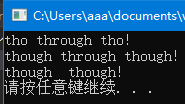## 练习9.44

#include <iostream>
#include <string>
#include <vector>
using namespace std;
void replace_string(string &s, const string &oldVal, const string &newVal)
{
int p = 0;
while ((p = s.find(oldVal, p)) != string::npos)
{
s.replace(p, oldVal.size(), newVal);
p += newVal.size();
}
}
int main()
{
string s = "tho thru tho!";
replace_string(s, "thru", "through");
cout << s << endl;
replace_string(s, "tho", "though");
cout << s << endl;
replace_string(s, "through", "");
cout << s << endl;
system("pause");
return 0;
}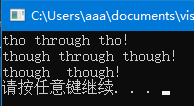## 练习9.45

#include <iostream>
#include <string>
#include <vector>
using namespace std;
void name_string(string &name, const string &prefix, const string &suffix)
{
name.insert(name.begin(), 1, ' ');
name.insert(name.begin(), prefix.begin(), prefix.end());
name.append(" ");
name.append(suffix.begin(), suffix.end());
}
int main()
{
string s = "James Bond";
name_string(s, "Mr.", "II");
cout << s << endl;
s = "M";
name_string(s, "Mrs.", "III");
cout << s << endl;
system("pause");
return 0;
}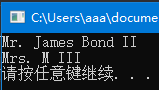## 练习9.46

#include <iostream>
#include <string>
#include <vector>
using namespace std;
void name_string(string &name, const string &prefix, const string &suffix)
{
name.insert(0, " ");
name.insert(0, prefix);
name.insert(name.size(), " ");
name.insert(name.size(), suffix);
}
int main()
{
string s = "James Bond";
name_string(s, "Mr.", "II");
cout << s << endl;
s = "M";
name_string(s, "Mrs.", "III");
cout << s << endl;
system("pause");
return 0;
}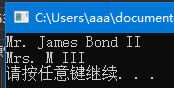## 练习9.47

find_first_of 在字符串中查找给定字符集合中任一字符首次出现的位置。若查找数字字符，则“给定字符集合”应包含所有10个数字；若查找字母，则要包含所有大小写字母—abcdefghijklmnopqrstuvwxyzABCDEFGHIJKLMNOQRSTUVWXYZ

#include <iostream>
#include <string>
#include <vector>
using namespace std;
void find_char(string &s, const string &chars)
{
cout << "在" << s << "中查找" << chars << "中字符" << endl;
string::size_type pos = 0;
while ((pos=s.find_first_of(chars, pos))!=string::npos)
{
cout << "pos: " << pos << ",char: " << s[pos] << endl;
pos++;
}
}
int main()
{
string s = "ab2c3d7R4E6";
cout << "查找所有数字" << endl;
find_char(s, "0123456789");
cout << endl << "查找所有字母" << endl;
find_char(s, "abcdefghijklmnopqrstuvwxyz" \
"ABCDEFGHIJKLMNOPQRSTUVWXYZ");
system("pause");
return 0;
}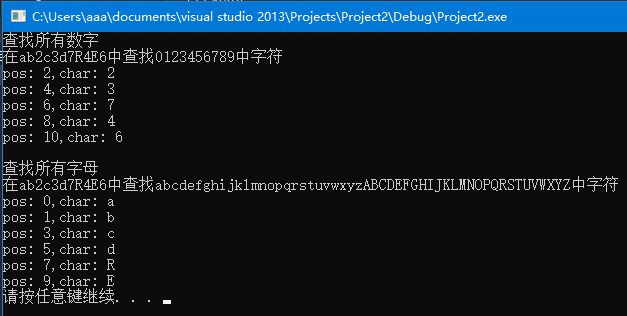find_first_not_of 查找第一个不在给定字符集合中出现的字符，若用它查找某类字符首次出现的位置，则应使用补集。若查找数字字符，则“给定字符集合”应包含10个数字之外的所有字符—abcdefghijklmnopqrstuvwxyzABCDEFGHIJKL MNOPQRSTUVWXYZ；若查找字母，则要包含所有非字母字符。

#include <iostream>
#include <string>
#include <vector>
using namespace std;
void find_not_char(string &s, const string &chars)
{
cout << "在" << s << "中查找不在" << chars << "中字符" << endl;
string::size_type pos = 0;
while ((pos=s.find_first_not_of(chars, pos))!=string::npos)
{
cout << "pos: " << pos << ",char: " << s[pos] << endl;
pos++;
}
}
int main()
{
string s = "ab2c3d7R4E6";
cout << "查找所有数字" << endl;
find_not_char(s, "abcdefghijklmnopqrstuvwxyz" \
"ABCDEFGHIJKLMNOPQRSTUVWXYZ");
cout << endl << "查找所有字母" << endl;
find_not_char(s, "0123456789");
system("pause");
return 0;
}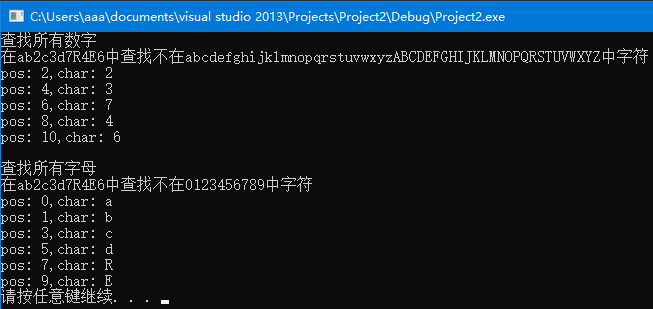## 练习9.48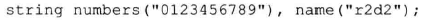s.find(args) 查找 sargs 第一次出现的位置，即第一个与 args 匹配的字符串的位置。args 是作为一个字符串整体在 s 中查找，而非一个字符集合在 s 中查找其中字符。因此，对325页给定的 namenumbers 值，在 numbers 中不存在与 name 匹配的字符串，find 会返回 npos

## 练习9.49

#include <iostream>
#include <string>
#include <vector>
#include <fstream>          // file I/O support
#include <cstdlib>          // support for exit()
using namespace std;
void find_longest_word(ifstream &in){
string s, longest_word;
int max_length = 0;
while (in >> s)
{
if (s.find_first_of("bdfghjklpqty") != string::npos)
continue;
cout << s << " ";
if (max_length < s.size()){
max_length = s.size();
longest_word = s;
}
}
cout << endl << "最长字符串：" << longest_word << endl;
}
int main()
{
char filename;
cout << "Enter name of data file: ";
cin.getline(filename, 20);
ifstream inFile;        // object for handling file input
inFile.open(filename);  // associate inFile with a file
if (!inFile.is_open())  // failed to open file
{
cout << "Could not open the file " << filename << endl;
cout << "Program terminating.\n";
// cin.get();    	// keep window open
exit(EXIT_FAILURE);
}
find_longest_word(inFile);
inFile.close();         // finished with the file

system("pause");
return 0;
}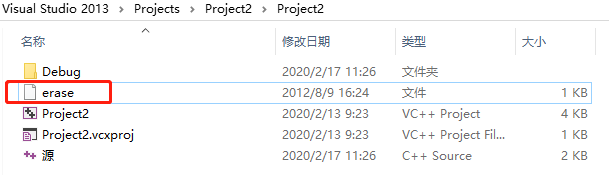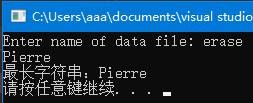## 练习9.50

#include <iostream>
#include <string>
#include <vector>
using namespace std;
int main()
{
vector<string> vs = { "123", "+456", "-789" };
int sum = 0;
for (auto iter = vs.begin(); iter != vs.end(); iter++){
sum += stoi(*iter);
}
cout << "和：" << sum << endl;

system("pause");
return 0;
}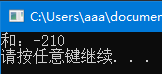#include <iostream>
#include <string>
#include <vector>
using namespace std;
int main()
{
vector<string> vs = { "12.3", "-4.56", "+7.8e-2" };
float sum = 0;
for (auto iter = vs.begin(); iter != vs.end(); iter++){
sum += stof(*iter);
}
cout << "和：" << sum << endl;

system("pause");
return 0;
}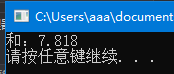## 练习9.51

1. 若首字符是数字，则为格式2，用 stoi 提取月份值，若月份值不合法，抛出异常，否则转到步骤6。
2. 若首字符不是数字，表明是格式1或3，首先提取月份值。
3. ds 开始的子串与月份简称进行比较，若均不等，抛出异常（若与简称不等，则不可能与全称相等）。
4. 若与第 i 个月简称相等，且下一个字符是合法间隔符，返回月份值。
5. 否则，检查接下来的子串是否与全称剩余部分相等，若不等，抛出异常；否则，返回月份值。
6. stoi 提取日期值和年份值，如需要，检查间隔符合法性。

data.h

#ifndef DATE_H_INCLUDED
#define DATE_H_INCLUDED
#include<iostream>
#include<string>
#include<stdexcept>
using namespace std;
class date{
public:
friend ostream& operator<<(ostream&, const date&);
date() = default;
date(string &ds);
unsigned y() const { return year; }
unsigned m() const { return month; }
unsigned d() const { return day; }
private:
unsigned year, month, day;
};

// 月份全称
const string month_name[] = { "January", "February", "March",
"April", "May", "June", "July", "August", "September",
"October", "November", "December" };

// 月份简写
const string month_abbr[] = { "Jan", "Feb", "Mar", "Apr", "May",
"Jun", "Jul", "Aug", "Sept", "oct", "Nov", "Dec" };

// 每月天数
const int days[] = { 31, 28, 31, 30, 31, 30, 31, 31, 30, 31, 30, 31 };

inline int get_month(string &ds, int &end_of_month){
int i, j;
for (i = 0; i < 12; i++){
// 检查每个字符是否与月份简写相等
for (j = 0; j < month_abbr[i].size(); j++)
if (ds[j] != month_abbr[i][j])	//不是此月简写
break;
if (j == month_abbr[i].size())	// 与简写匹配
break;
}
if (i == 12)		// 与所有月份名都不相同
throw invalid_argument("不是合法月份名");
if (ds[j] == ' '){		//空白符，仅是月份简写
end_of_month = j + 1;
return i + 1;
}

for (; j < month_name[i].size(); j++)
if (ds[j] != month_name[i][j])
break;
if (j == month_name[i].size() && ds[j] == ' '){	//月份全称
end_of_month = j + 1;
return i + 1;
}
throw invalid_argument("不是合法月份名");
}

inline int get_day(string&ds, int month, int &p){
size_t q;

int day = stoi(ds.substr(p), &q);	// 从p开始的部分转换为日期值
if (day<1 || day>days[month])
throw invalid_argument("不是合法日期值");
p += q;//移动到日期值之后
return day;
}

inline int get_year(string &ds, int &p){
size_t q;
int year = stoi(ds.substr(p), &q);	// 从p开始的部分转换为年
if (p + q<ds.size())
throw invalid_argument("非法结尾内容");
return year;
}

date::date(string &ds){
int p;
size_t q;
if ((p = ds.find_first_of("0123456789")) == string::npos)
throw invalid_argument("没有数字，非法日期");
if (p>0){		// 月份名格式
month = get_month(ds, p);
day = get_day(ds, month, p);
if (ds[p] != ' ' && ds[p] != ',')
throw invalid_argument("非法间隔符");
p++;
year = get_year(ds, p);
}
else{			// 月份值格式
month = stoi(ds, &q);
p = q;
if (month<1 || month >12)
throw invalid_argument("不是合法月份值");
if (ds[p++] != '/')
throw invalid_argument("非法间隔符");
day = get_day(ds, month, p);
if (ds[p++] != '/')
throw invalid_argument("非法间隔符");
year = get_year(ds, p);
}
}

ostream & operator<<(ostream& out, const date& d){
out << d.y() << "年" << d.m() << "月" << d.d() << "日" << endl;
return out;
}
#endif	// DATE_H_INCLUDED


main.cpp

#include <iostream>
#include <string>
#include "data.h"
using namespace std;
int main()
{
string dates[] = { "Jan 1, 2014", "February 1 2014", "3/1/2014", "3 1 2014"
//"Jcn 1，2014"，
//"Janvary 1，2014"，
//"Jan 32，2014"，
//"Jan 1/2014"，
};
try{
for (auto ds : dates){
date dl(ds);
cout << dl;
}
}
catch (invalid_argument e){
cout << e.what() << endl;
}
system("pause");
return 0;
}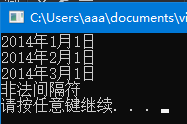## 练习9.52

1. 读入了一个运算数 v
a）若栈空或栈顶是左括号，则 v 是第一个运算数，直接压栈即可。
b）否则，v 前必须是一个运算符，再之前是另一个运算数 v'，从栈顶弹出这两项，将计算结果压栈即可；否则，就抛出一个“缺少运算符”异常。
2. 读入了一个左括号，直接压栈。
3. 读入了一个运算符，
a）若栈空或栈顶不是一个运算数，则抛出一个“缺少运算数”异常。注意，若运算符之前是一个右括号，之前也已处理完毕，栈顶是其计算结果，仍应该是运算数，不影响此逻辑。
b）否则，运算符压栈。
4. 读入了一个右括号，
a）若栈空，表明之前没有与之配对的左括号，抛出“未匹配右括号”异常。
b）若栈顶是左括号，表明括号对之间无表达式，抛出“空括号”异常。
c）若栈顶不是运算数，表明括号内缺少一个运算数，抛出一个异常。
d）弹出此运算数 v，若栈空或栈顶不是左括号，仍抛出“未匹配右括号”异常；否则弹出左括号，把 v作为新运算数，执行1中的逻辑。
5. 以上均不是，则出现了非法输入，会在转换为数值时产生异常。
6. 当字符串处理完毕后，判断栈中是否有且仅有一个运算数，若是，此值即为表达式运算结果，输出它；否则，表达式非法。

#include<iostream>
#include<string>
#include<deque>
#include<stack>
#include<stdexcept>
using namespace std;
//表示栈中对象的不同类型
enum obj_type{ LP, RP, ADD, SUB, VAL };
struct obj{
obj(obj_type type, double val = 0){ t = type; v = val; }
obj_type t;
double v;
};
inline void skipws(string &exp, size_t &p){
p = exp.find_first_not_of(" ", p);
}
inline void new_val(stack<obj> &so, double v){
if (so.empty() || so.top().t == LP){	//空栈或左括号
so.push(obj(VAL, v));
//cout << "push" << v << endl;
}
else if(so.top().t == ADD || so.top().t == SUB){
//之前是运算符
obj_type type = so.top().t;
so.pop();
/*
cout << "pop+" << endl;
else
cout << "pop-" << endl;
*/
// cout << "pop" << so.top().v << endl;
// 执行加减法
v += so.top().v;
else
v = so.top().v - v;
so.pop();
so.push(obj(VAL, v));			//运算结果压栈
//cout <<"push"<< v<< endl；
}
else
throw invalid_argument("缺少运算符");
}

int main()
{
stack<obj> so;
string exp;
size_t p = 0, q;
double v;
cout << "请输入表达式：";
getline(cin, exp);
while (p < exp.size()){
skipws(exp, p);					//跳过空格
if (exp[p] == '('){				//左括号直接压栈
so.push(obj(LP));
p++;
//cout <<"push LP"<< endl;
}
else if (exp[p] == '+' || exp[p] == '-'){
//新运算符
if (so.empty() || so.top().t != VAL)
//空栈或之前不是运算数
throw invalid_argument("缺少运算数");
if (exp[p] == '+')			//运算符压栈
else
so.push(obj(SUB));
p++;
//cout <<"push"<< exp[p-1]<< endl；
}
else if (exp[p] == ')'){		//右括号
p++;
if (so.empty())				//之前无配对的左括号
throw invalid_argument("未匹配右括号");
if (so.top().t == LP)		//一对括号之间无内容
throw invalid_argument("空括号");
if (so.top().t == VAL){		//正确：括号内运算结果
v = so.top().v;
so.pop();
//cout <<"pop"<<v<< endl；
if (so.empty() || so.top().t != LP)
throw invalid_argument("未匹配右括号");
so.pop();
//cout <<"pop LP"<<endl；
new_val(so, v);			//与新运算数逻辑一致
}
else
//栈顶必定是运算符
throw invalid_argument("缺少运算数");
}
else{			//应该是运算数
v = stod(exp.substr(p), &q);
p += q;
new_val(so, v);
}
}
if (so.size() != 1 || so.top().t != VAL)
throw invalid_argument("非法表达式");
cout << so.top().v << endl;

system("pause");
return 0;
}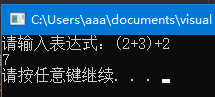### 最后两道题看的大佬书籍上的写法，还在研究中。

©️2019 CSDN 皮肤主题: 酷酷鲨 设计师: CSDN官方博客